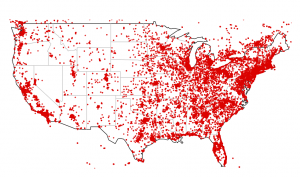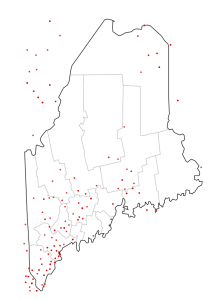Want to share your content on R-bloggers? click here if you have a blog, or here if you don't.

Since F-Secure was #spiffy enough to provide us with GeoIP data for mapping the scope of the ZeroAccess botnet, I thought that some aspiring infosec data scientists might want to see how to use something besides Google Maps & Google Earth to view the data.

If you look at the CSV file, it’s formatted as such (this is a small portion…the file is ~140K lines):

``` CL,"-34.9833","-71.2333" PT,"38.679","-9.1569" US,"42.4163","-70.9969" BR,"-21.8667","-51.8333" ```

While that’s useful, we don’t need quotes and a header would be nice (esp for some of the tools I’ll be showing), so a quick cleanup in `vi` gives us:

``` Code,Latitude,Longitude CL,-34.9833,-71.2333 PT,38.679,-9.1569 US,42.4163,-70.9969 BR,-21.8667,-51.8333 ```

With just this information, we can see how much of the United States is covered in ZeroAccess with just a few lines of R:

``` # read in the csv file bots = read.csv("ZeroAccessGeoIPs.csv")   # load the maps library library(maps)   # draw the US outline in black and state boundaries in gray map("state", interior = FALSE) map("state", boundary = FALSE, col="gray", add = TRUE)   # plot the latitude & longitudes with a small dot points(x=bots\$Longitude,y=bots\$Latitude,col='red',cex=0.25) ```

Can you pwn me now?Click for larger map

If you want to see how bad your state is, it’s just as simple. Using my state (Maine) it’s just a matter of swapping out the `map` statements with more specific data:

``` bots = read.csv("ZeroAccessGeoIPs.csv") library(maps)   # draw Maine state boundary in black and counties in gray map("state","maine",interior=FALSE) map("county","maine",boundary=FALSE,col="gray",add=TRUE)   points(x=bots\$Longitude,y=bots\$Latitude,col='red',cex=0.25) ```

We’re either really tech/security-savvy or don’t do much computin’ up hereClick for larger map

Because of the way the `maps` library handles geo-plotting, there are points outside the actual map boundaries.

You can even get a quick and dirty geo-heatmap without too much trouble:

``` bots = read.csv("ZeroAccessGeoIPs.csv")   # load the ggplot2 library library(ggplot2)   # create an plot object for the heatmap zeroheat <- qplot(xlab="Longitude",ylab="Latitude",main="ZeroAccess Botnet",geom="blank",x=bots\$Longitude,y=bots\$Latitude,data=bots) + stat_bin2d(bins =300,aes(fill = log1p(..count..)))   # display the heatmap zeroheat ```Click for larger map

Try playing around with the `bins` to see how that impacts the plots (the `stat_bin2d(…)` divides the “map” into “buckets” (or bins) and that informs plot how to color code the output).

If you were to pre-process the data a bit, or craft some ugly R code, a more tradtional choropleth can easily be created as well. The interesting part about using a non-boundaried plot is that this ZeroAccess network almost defines every continent for us (which is kinda scary).

That’s just a taste of what you can do with just a few, simple lines of R. If I have some time, I’ll toss up some examples in Python as well. Definitely drop a note in the comments if you put together some #spiffy visualizations with the data they provided.